# What is the difference between an ingredient "piece" and a recipe "portion".

## When you define ingredient- or recipe packages, you can set a certain package as a piece (ingredient) or portion (recipe). This article explains the difference and similarities between these two.

When creating a recipe, it is often easier to define some ingredients or subrecipes in a unitary amount instead of in weight or volume. The unit in our software to define them in a unitary amount is "piece" for ingredients and "portions" for recipes (when used as sub-recipes).

### Ingredient

If you want to use an ingredient in a recipe with the "piece" unit, there are two different ways to go about it:

• Create a package defined "pieces" as a unit e.g your package is 12 "pieces" of egg. A drawback to this approach is that the weight or volume of this ingredient is not taken into account with the automatically calculated weight of a recipe. Also if you want to calculate the nutritional values of recipes you won't be able to, because you don't know the volume or weight of the ingredients only defined as pieces.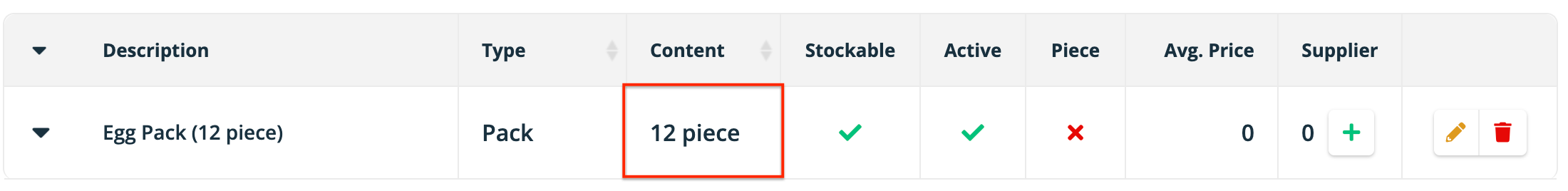• You create a package in a mass or volume unit e.g 200 ml and define it as the piece. This way when you use 3 pieces of that ingredient it is the same thing as using 600 ml. With this approach, you can use both pieces and mass/volume units and the ingredient's weight will be taken into consideration for the recipe's calculated weight and you will be able to calculate nutritional values.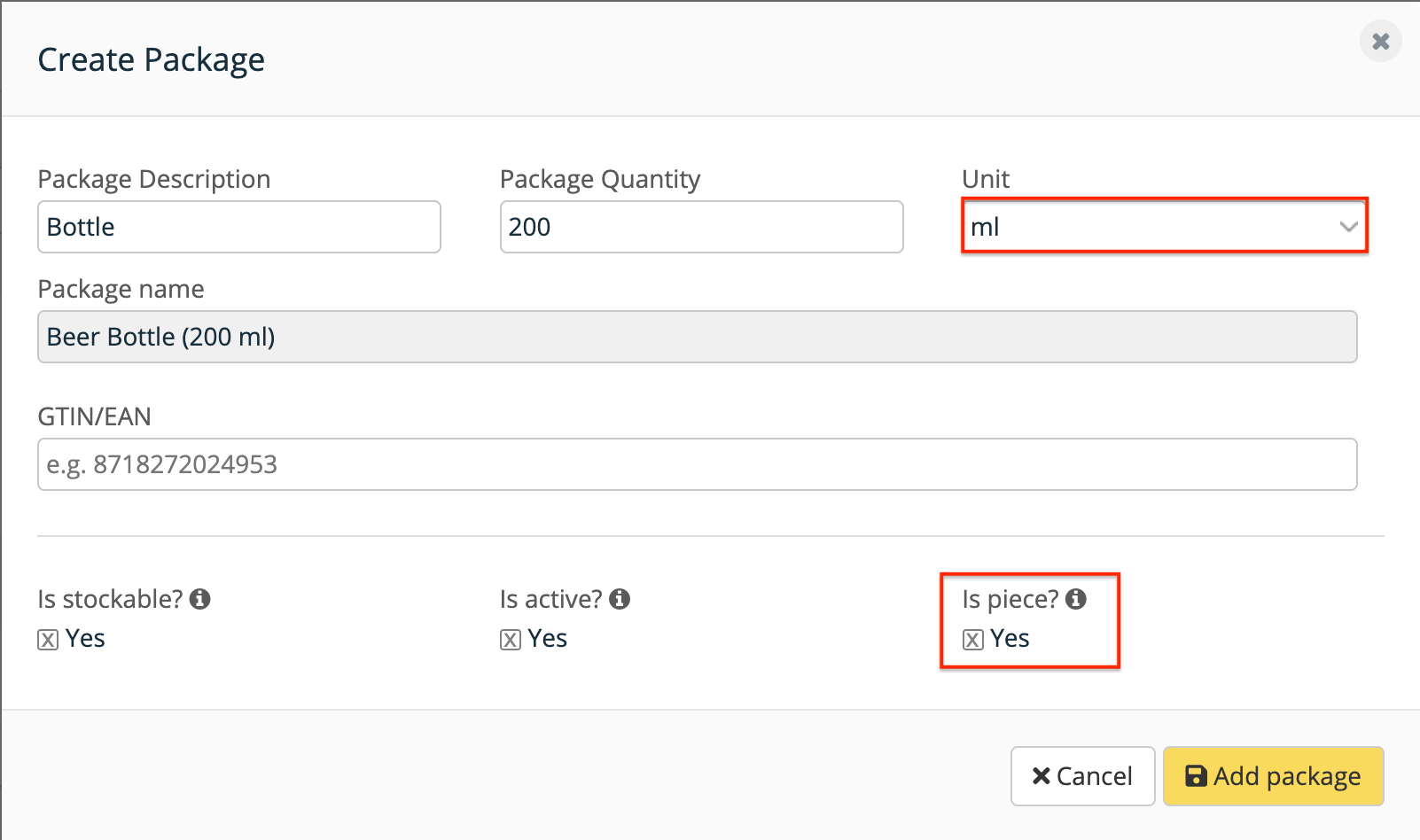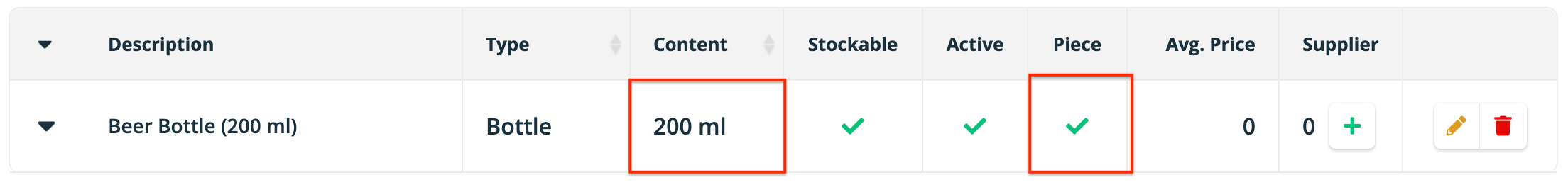### Recipe

A recipe always has a portion defined. There are two different ways to do it

• The manually set or calculated volume or weight will be divided by the number of portions. This will be the volume of weight per portion.
• If the amount of portions is not set we will consider the whole recipe as 1 portion

In the screenshot below you can see the recipe doesn't have the manual weight set so we fall back to the calculated (the leftmost numbers in the panel are the most important and shows the portion size we will use).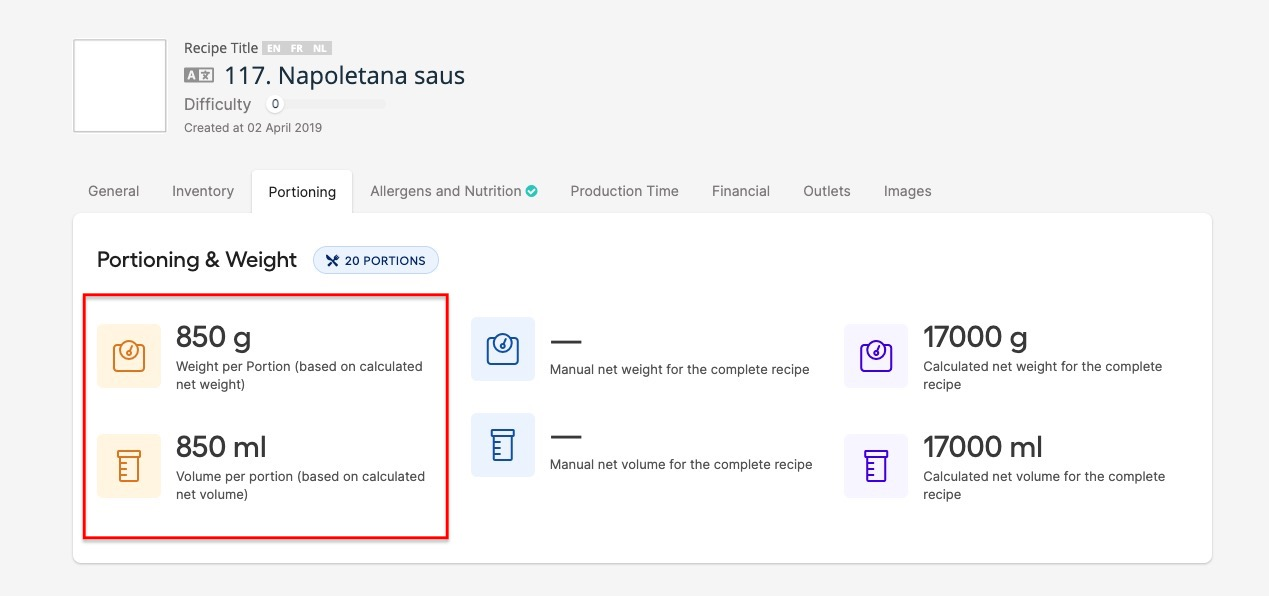### Stockable Recipe

For a stockable recipe, since it also has packages, you can define a package as a "Portion". It behaves exactly as the ingredient so whenever a recipe uses 'portions' of sub-recipes we will take the weight of the package defined as "Portion".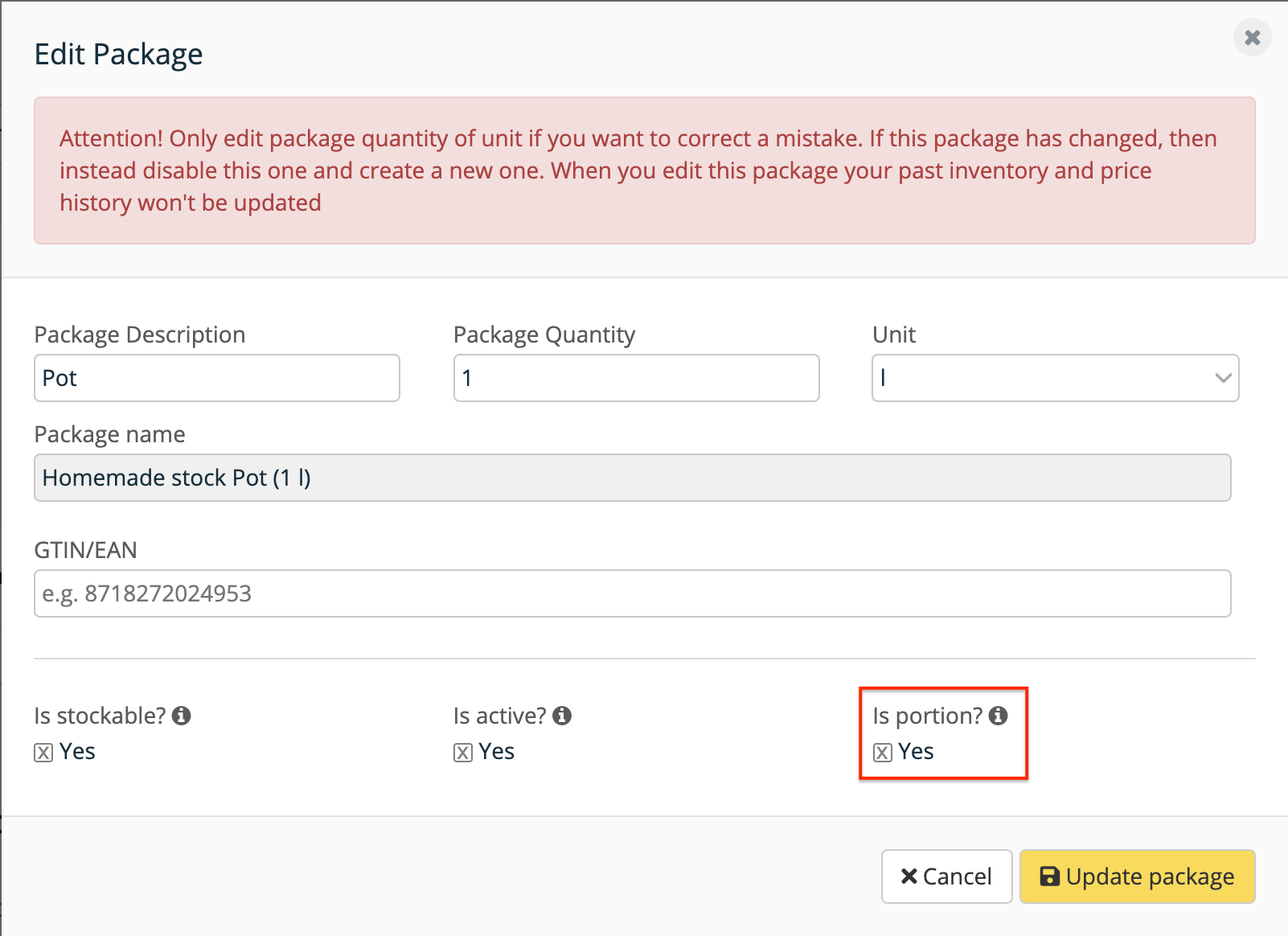The difference when comparing with an ingredient is that if a package is not set as a portion we will fallback to:

1. First: Manually inputted recipe weight divided by the number of portions.
2. Second: Calculated recipe's weight divided by portion.

Another difference is that inventory events of recipes e.g sales, waste is always calculated based on their portion, so in the example above if we sell 2 portions of Homemade stock, we will withdraw 2 liters.